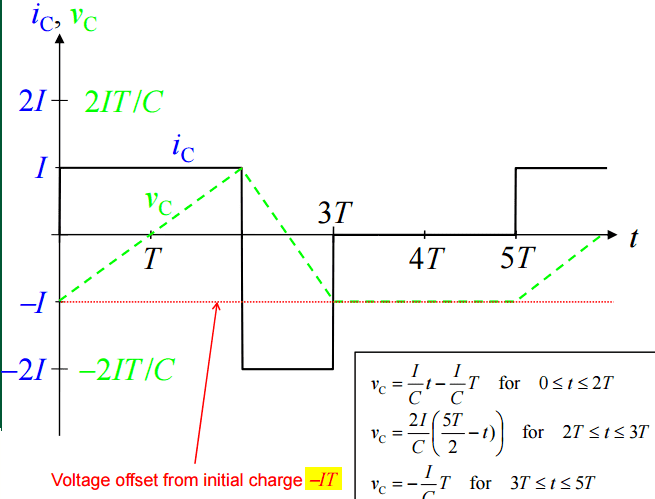# Capacitor Charge/Discharge boundary conditions for time

Member advised to use the homework template for posts in the homework sections of PF.

## Homework Equations

3. The Attempt at a Solution [/B]I know dV=1/C∫idt and that we integrate the voltage from V to V0. What I don't get are the boundary conditions for t - How do we get what we get in the parenthesis? My closest assumption is that the t/T values refer to the areas described by the voltage graph - triangles and rectangles. I have noticed that the we section the period every time there's a change in current, and therefore, in voltage (0<t<2T, 2T<t<3T, etc.)

Also what use am I supposed to make of Q=-IT? From this equation and the first one we get i=dQ/dt, but so what?

Grinkle
Gold Member
Not sure I understand your question.

I see a piece-wise definition of v(t) on the graphic in the lower right. Is that what you are asking about?

Yes. I don't get why we have what we have in the parenthesis - I thought for 0<t<2T we'd have I(2T-0)/C but we have I(t-T)/C instead. Same goes for the rest.

NascentOxygen
Staff Emeritus
•